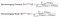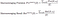# Multi-label classification for threat detection (part 1)

At GumGum, providing a brand safe environment for our advertisers is of utmost priority. In order to achieve this, the publisher’s inventory is scanned through to avoid ad misplacement. As CV scientists we build systems that can detect and classify threats if present in the publisher’s inventory, which could be images and/or videos. In order to detect and classify these threats, convolutional neural network based image classification algorithms are employed. A conventional multiclass image classifier can often times work well when an object under consideration is the only one in the image or occupies a large enough area of the image. Unfortunately this is far from reality: the images our publishers have generally contain multiple objects.

For example, consider the following image. A multiclass classifier model classifies this as a safe image because the salient object is a baked dish. However, the image also contain an alcoholic beverage in the background, which is considered unsafe by certain advertisers.

In order to alleviate this problem, we build and evaluate a multi-label classifier. In the following section, the specifics of the dataset used for proof of concept, modelling and the evaluation metrics are explained. Another motivation for using a multilabel classifier was a simple top 2 accuracy on the multiclass classifier resulted in a 14% increase in overall accuracy, which can be extracted using the multilabel classifier.

Data :
The dataset used here is the amazon planet understanding dataset which consists of satellite imagery with various atmospheric conditions. The aim is to identify deforestation in these images effectively. The data distribution of the 17 classes is as shown.

Minimal image preprocessing like normalization and data augmentation like random horizontal flipping is performed. The training setup involved 4 NVIDIA GeForce GTX 1080 GPUs and it took approximately an hour to train this network since the data did not involve significant complexity.

Model :

EfficientNet is used as the network for this multilabel classifier. Convolutional neural networks typically can be designed by three types of scaling -

• Depth scaling — It involves vertical scaling by increasing the number of layers in the network.
• Width scaling — It involves horizontal scaling by increasing the number of channels in layers of the network.
• Resolution scaling — It involves increasing the image resolution being accepted as input into the network.

EfficientNet is based on a network derived from a neural architecture search and novel compound scaling method is applied to iteratively build more complex network which achieves state of the art accuracy on multiclass classification tasks. Compound scaling refers to increasing the network dimensions in all three scaling formats using a novel strategy.

Unlike for a multiclass classification problem which uses a softmax layer at the output, we will be using a sigmoid layer. Softmax is often used to map the non-normalized output of a multiclass network to a probability distribution over predicted output classes. The maximum probability class is then chosen as the final class. This is exactly what we want to avoid in our multilabel classification implementation. In this case all classes are mutually exclusive and the probability of occurrence of one class is independent of the occurrence of another class. This can be modelled by using a sigmoid activation at the output layer. A binary cross entropy loss function is used for optimization in the multilabel setup. With the right set of hyperparameters this model is trained and evaluated on the amazon dataset whose performance is detailed in the following section.

Evaluation metrics and results :

Evaluation of multiclass classification can be done using simple accuracy metrics, that is a correct prediction is an exact match between the prediction and ground truth which is given by -

But in a multilabel setting this would be a harsh evaluation metric, as a model getting a subset of the positive classes right performs better than a model getting no classes right. In this regard, micro averaging and macro averaging are used where we average out each class.The true positives, false positives and false negatives are summed up.Class-wise precision and recall are averaged to provide an overall system performance.

These measures can be used to calculate the F-beta score. Another label measure that provides good insights into the performance of the multilabel system is the hamming score based on the hamming distance. It penalizes for every label that the prediction gets wrong which is essentially what we want.

Compared to Hamming score, F-beta enforces a better balance between relevant and irrelevant labels. Therefore , F-beta is more suitable for multilabel problems in which irrelevant labels prevail. We present results on both measures.

The state of the art method involves using an ensemble of classifiers and using ridge classifiers on the output labels to correctly determine the classes. This significantly increases the computational requirements and hence we stick to using EfficientNet as a single network multilabel classifier. Using the simplest EfficientNet-B0 model we achieve comparable performance to the SOTA method.

In a future blog post results on model trained on our dataset will be presented and benchmarked against the previous models used in production.

We’re always looking for new talent! View jobs.

Written by